# Time Series Prediction Using LSTM Deep Neural Networks

#### Jakob Aungiers

1st September 2018

This article focuses on using a Deep LSTM Neural Network architecture to provide multidimensional time series forecasting using Keras and Tensorflow - specifically on stock market datasets to provide momentum indicators of stock price.

The code for this framework can be found in the following GitHub repo (it assumes python version 3.5.x and the requirement versions in the requirements.txt file. Deviating from these versions might cause errors): https://github.com/jaungiers/LSTM-Neural-Network-for-Time-Series-Prediction

The following article sections will briefly touch on LSTM neuron cells, give a toy example of predicting a sine wave then walk through the application to a stochastic time series. The article assumes a basic working knowledge of simple deep neural networks.

## What Are LSTM Neurons?

One of the fundamental problems which plagued traditional neural network architectures for a long time was the ability to interpret sequences of inputs which relied on each other for information and context. This information could be previous words in a sentence to allow for a context to predict what the next word might be, or it could be temporal information of a sequence which would allow for context on the time based elements of that sequence.

Simply put, traditional neural networks take in a stand-alone data vector each time and have no concept of memory to help them on tasks that need memory.

An early attempt to tackle this was to use a simple feedback type approach for neurons in the network where the output was fed-back into the input to provide context on the last seen inputs. These were called Recurrent Neural Networks (RNNs). Whilst these RNNs worked to an extent, they had a rather large downfall that any significant uses of them lead to a problem called the Vanishing Gradient Problem. We will not expand on the vanishing gradient issue any further than to say that RNNs are poorly suited in most real-world problems due to this issue, hence, another way to tackle context memory needed to be found.

This is where the Long Short Term Memory (LSTM) neural network came to the rescue. Like RNN neurons, LSTM neurons kept a context of memory within their pipeline to allow for tackling sequential and temporal problems without the issue of the vanishing gradient affecting their performance.

Many research papers and articles can be found online which discuss the workings of LSTM cells in great mathematical detail. In this article however we will not discuss the complex workings of LSTMs as we are more concerned about their use for our problems.

For context, below is a diagram of the typical inner workings of an LSTM neuron. It consists of several layers, and pointwise operations which act as gates for data input, output and forget which feed the LSTM cell state. This cell state is what keeps the long-term memory and context across the network and inputs.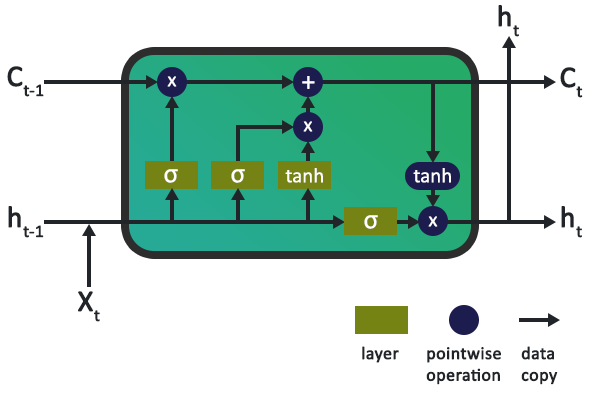## A Simple Sine Wave Example

To demonstrate the use of LSTM neural networks in predicting a time series let us start with the most basic thing we can think of that's a time series: the trusty sine wave. And let us create the data we will need to model many oscillations of this function for the LSTM network to train over.

The data provided in the code's data folder contains a sinewave.csv file we created which contains 5001 time periods of a sine wave with amplitude and frequency of 1 (giving an angular frequency of 6.28) and a time delta of 0.01. The result of this, when plotted looks like this:Dataset for a sinewave

Now that we have the data, what are we actually trying to achieve? Well, simply we want the LSTM to learn the sine wave from a set window size of data that we will feed it and hopefully we can ask the LSTM to predict the next N-steps in the series and it will keep outputting a sine wave.

We will start by transforming and loading the data from the CSV file to a pandas dataframe which will then be used to output a numpy array that will feed the LSTM. The way Keras LSTM layers work is by taking in a numpy array of 3 dimensions (N, W, F) where N is the number of training sequences, W is the sequence length and F is the number of features of each sequence. We chose to go with a sequence length (read window size) of 50 which allows for the network so get glimpses of the shape of the sine wave at each sequence and hence will hopefully teach itself to build up a pattern of the sequences based on the prior window received.

The sequences themselves are sliding windows and hence shift by 1 each time, causing a constant overlap with the prior windows. A typical training window of sequence length 50, when plotted, is shown below: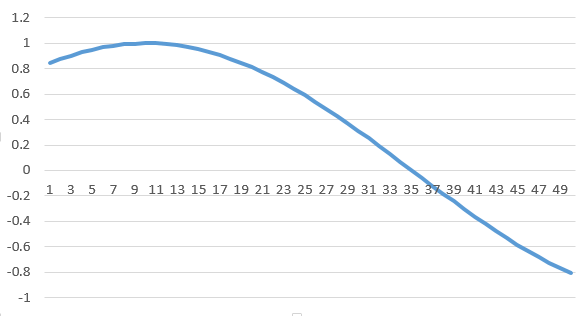Sinewave dataset training window

For loading this data we created a DataLoader class in our code to provide an abstraction for the data loading layer. You will notice that upon initialization of a DataLoader object, the filename is passed in, along with a split variable which determines the percentage of the data to use for training vs. testing and a columns variable which allows for selecting one or more columns of data for single dimensional or multidimensional analysis.

class DataLoader():

def __init__(self, filename, split, cols):
i_split = int(len(dataframe) * split)
self.data_train = dataframe.get(cols).values[:i_split]
self.data_test  = dataframe.get(cols).values[i_split:]
self.len_train  = len(self.data_train)
self.len_test   = len(self.data_test)
self.len_train_windows = None

def get_train_data(self, seq_len, normalise):
data_x = []
data_y = []
for i in range(self.len_train - seq_len):
x, y = self._next_window(i, seq_len, normalise)
data_x.append(x)
data_y.append(y)
return np.array(data_x), np.array(data_y)



After we have a data object which allows for us to load the data we will need to build the deep neural network model. Again for abstraction our code framework uses a Model class alongside a config.json file to easily build an instance of our model given a required architecture and hyperparameters stored in the config file. The main function which builds our network is the build_model() functions that takes in the parsed configs file.

This function code can be seen below and can easily be extended for future use on more complex architectures.

class Model():

def __init__(self):
self.model = Sequential()

def build_model(self, configs):
timer = Timer()
timer.start()

for layer in configs['model']['layers']:
neurons = layer['neurons'] if 'neurons' in layer else None
dropout_rate = layer['rate'] if 'rate' in layer else None
activation = layer['activation'] if 'activation' in layer else None
return_seq = layer['return_seq'] if 'return_seq' in layer else None
input_timesteps = layer['input_timesteps'] if 'input_timesteps' in layer else None
input_dim = layer['input_dim'] if 'input_dim' in layer else None

if layer['type'] == 'dense':
if layer['type'] == 'lstm':
if layer['type'] == 'dropout':

self.model.compile(loss=configs['model']['loss'], optimizer=configs['model']['optimizer'])

print('[Model] Model Compiled')
timer.stop()



With the data loaded and the model built we can now progress onto training the model with our training data. For this we create a separate run module which will utilize our Model and DataLoader abstractions to combine them for training, output and visualizations.

Below is the general run thread code to train our model.

configs = json.load(open('config.json', 'r'))

os.path.join('data', configs['data']['filename']),
configs['data']['train_test_split'],
configs['data']['columns']
)

model = Model()
model.build_model(configs)
x, y = data.get_train_data(
seq_len = configs['data']['sequence_length'],
normalise = configs['data']['normalise']
)

model.train(
x,
y,
epochs = configs['training']['epochs'],
batch_size = configs['training']['batch_size']
)

x_test, y_test = data.get_test_data(
seq_len = configs['data']['sequence_length'],
normalise = configs['data']['normalise']
)



For output we will run two types of predictions: the first will be predicting in a point-by-point way, that is we are only predicting a single point ahead each time, plotting this point as a prediction, then taking the next window along with the full testing data and predicting the next point along once again.

The second prediction we will do is to predict a full sequence, by this we only initialize a training window with the first part of the training data once. The model then predicts the next point and we shift the window, as with the point-by-point method. The difference is we then predict using the data that we predicted in the prior prediction. In the second step this will mean only one data point (the last point) will be from the prior prediction. In the third prediction the last two data points will be from prior predictions and so forth. After 50 predictions our model will subsequently be predicting on its own prior predictions. This allows us to use the model to forecast many time steps ahead, but as it is predicting on predictions which can then in turn be based on predictions this will increase the error rate of the predictions the further ahead we predict.

Below we can see the code and respective outputs for both the point-by-point predictions and the full sequence predictions.

def predict_point_by_point(self, data):
#Predict each timestep given the last sequence of true data, in effect only predicting 1 step ahead each time
predicted = self.model.predict(data)
predicted = np.reshape(predicted, (predicted.size,))
return predicted

def predict_sequence_full(self, data, window_size):
#Shift the window by 1 new prediction each time, re-run predictions on new window
curr_frame = data
predicted = []
for i in range(len(data)):
predicted.append(self.model.predict(curr_frame[newaxis,:,:])[0,0])
curr_frame = curr_frame[1:]
curr_frame = np.insert(curr_frame, [window_size-2], predicted[-1], axis=0)
return predicted

predictions_pointbypoint = model.predict_point_by_point(x_test)
plot_results(predictions_pointbypoint, y_test)

predictions_fullseq = model.predict_sequence_full(x_test, configs['data']['sequence_length'])
plot_results(predictions_fullseq, y_test)Sinewave point-by-point prediction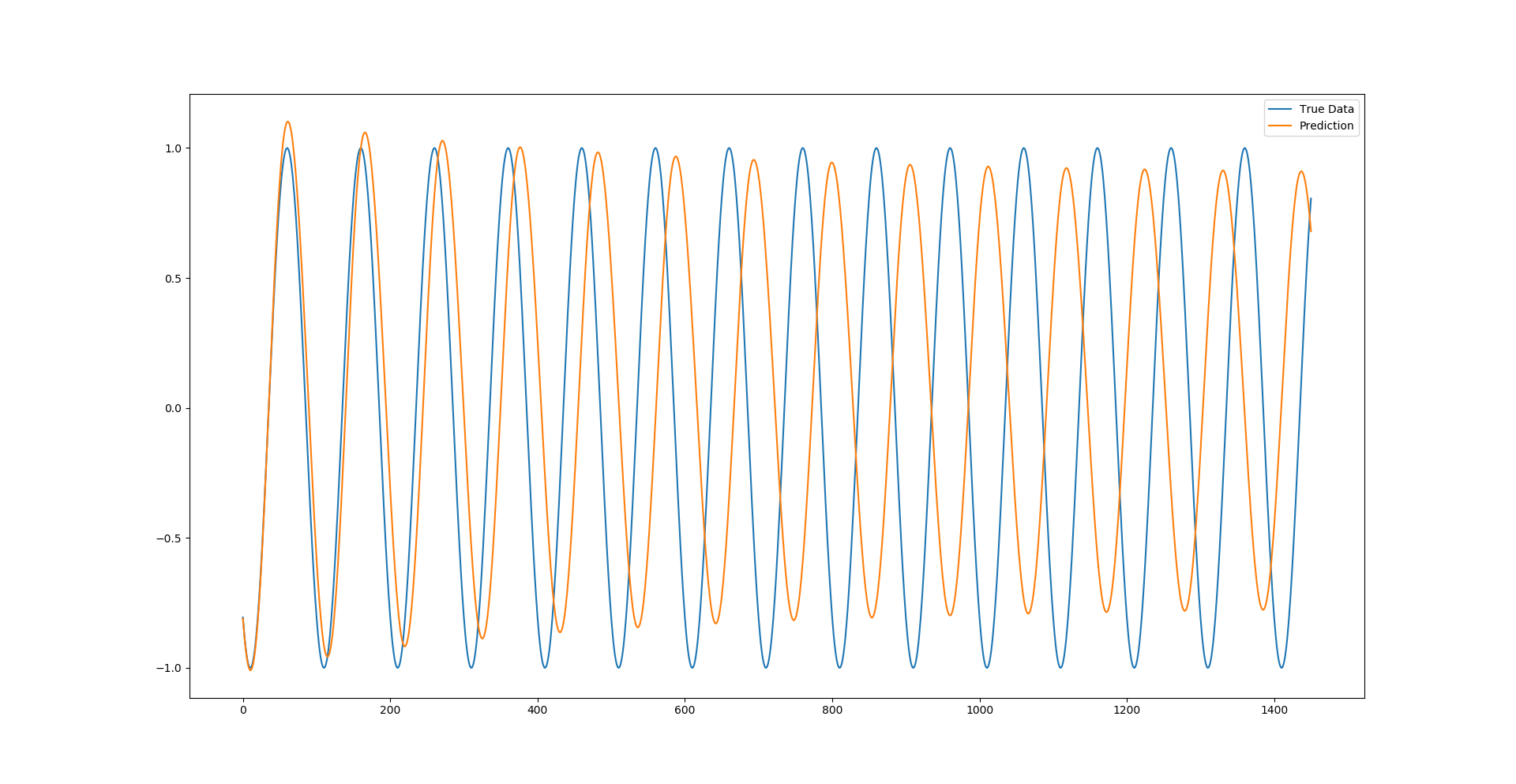Sinewave full sequence prediction

For reference the network architecture and hyperparameters used for the sinewave example can be seen in the below config file.

{
"data": {
"filename": "sinewave.csv",
"columns": [
"sinewave"
],
"sequence_length": 50,
"train_test_split": 0.8,
"normalise": false
},
"training": {
"epochs": 2,
"batch_size": 32
},
"model": {
"loss": "mse",
"layers": [
{
"type": "lstm",
"neurons": 50,
"input_timesteps": 49,
"input_dim": 1,
"return_seq": true
},
{
"type": "dropout",
"rate": 0.05
},
{
"type": "lstm",
"neurons": 100,
"return_seq": false
},
{
"type": "dropout",
"rate": 0.05
},
{
"type": "dense",
"neurons": 1,
"activation": "linear"
}
]
}
}



Overlaid with the true data we can see that with just 1 epoch and a reasonably small training set of data the LSTM deep neural network has already done a pretty good job of predicting the sine function.

You can see that as we predict more and more into the future the error margin increases as the errors in the prior predictions are amplified more and more when they are used for future predictions. As such we see that in the full sequence example, the further into the future we predict the less accurate the frequency and amplitude of the predictions is compared to the true data. However as the sin function is a very easy oscillating function with zero noise it can still predict it to a good degree without overfitting - this is important, as we could easily overfit the model by increasing the epochs and taking out the dropout layers to make it almost perfectly accurate on this training data, which is of the same pattern as the test data, but for other real-world examples overfitting the model onto the training data would cause the test accuracy to plummet as the model would not be generalizing.

In the next step we will try to use the model on such real-world data to see the effects.

## The Not-So-Simple Stock Market

We predicted a several hundred time steps of a sine wave on an accurate point-by-point basis. So we can now just do the same on a stock market time series and make immediate profit, right? Unfortunately in the real-world this is not quite that simple.

Unlike a sinewave, a stock market time series is not any sort of specific static function which can be mapped. The best property to describe the motion of a stock market time series would be a random walk. As a stochastic process, a true random walk has no predictable patterns and so attempting to model it would be pointless. Fortunately there are on-going arguments by many sides to say that a stock market isn't a pure stochastic process, which allows us to theorize that the time series may well have some kind of hidden pattern. And it is these hidden patterns that LSTM deep networks are prime candidates to predict.

The data this example will be using is the sp500.csv file in the data folder. This file contains the Open, High, Low, Close prices as well as the daily Volume of the S&P 500 Equity Index from January 2000 to September 2018.

In the first instance we will only create a single dimensional model using the Close price only. Adapting the config.json file to reflect the new data we will keep most of the parameters the same. One change which is needed however is that, unlike the sinewave which only had numerical ranges between -1 to +1 the close price is a constantly moving absolute price of the stock market. This means that if we tried to train the model on this without normalizing it, it would never converge.

To combat this we will take each n-sized window of training/testing data and normalize each one to reflect percentage changes from the start of that window (so the data at point i=0 will always be 0). We'll use the following equations to normalize and subsequently de-normalize at the end of the prediction process to get a real world number out of the prediction:

n = normalized list [window] of price changes
p = raw list [window] of adjusted daily return prices

Normalization:

De-Normalization:

We have added the normalise_windows() function to our DataLoader class to do this transformation, and a Boolean normalise flag is contained in the config file which denotes the normalization of these windows.

def normalise_windows(self, window_data, single_window=False):
'''Normalise window with a base value of zero'''
normalised_data = []
window_data = [window_data] if single_window else window_data
for window in window_data:
normalised_window = []
for col_i in range(window.shape):
normalised_col = [((float(p) / float(window[0, col_i])) - 1) for p in window[:, col_i]]
normalised_window.append(normalised_col)
# reshape and transpose array back into original multidimensional format
normalised_window = np.array(normalised_window).T
normalised_data.append(normalised_window)
return np.array(normalised_data)



With the windows normalized, we can now run the model in the same way that we ran it against out sinewave data. We have however made an important change when running this data; instead of using our framework's model.train() method, we are instead using the model.train_generator() method which we have created. We are doing this because we have found that it is easy to run out of memory when trying to train large datasets, as the model.train() function loads the full dataset into memory, then applies the normalizations to each window in-memory, easily causing a memory overflow. So instead we utilized the fit_generator() function from Keras to allow for dynamic training of the dataset using a python generator to draw the data, which means memory utilization will be minimized dramatically. The code below details the new run thread for running three types of predictions (point-by-point, full sequence and multiple sequence).

configs = json.load(open('config.json', 'r'))

os.path.join('data', configs['data']['filename']),
configs['data']['train_test_split'],
configs['data']['columns']
)

model = Model()
model.build_model(configs)
x, y = data.get_train_data(
seq_len = configs['data']['sequence_length'],
normalise = configs['data']['normalise']
)

# out-of memory generative training
steps_per_epoch = math.ceil((data.len_train - configs['data']['sequence_length']) / configs['training']['batch_size'])
model.train_generator(
data_gen = data.generate_train_batch(
seq_len = configs['data']['sequence_length'],
batch_size = configs['training']['batch_size'],
normalise = configs['data']['normalise']
),
epochs = configs['training']['epochs'],
batch_size = configs['training']['batch_size'],
steps_per_epoch = steps_per_epoch
)

x_test, y_test = data.get_test_data(
seq_len = configs['data']['sequence_length'],
normalise = configs['data']['normalise']
)

predictions_multiseq = model.predict_sequences_multiple(x_test, configs['data']['sequence_length'], configs['data']['sequence_length'])
predictions_fullseq = model.predict_sequence_full(x_test, configs['data']['sequence_length'])
predictions_pointbypoint = model.predict_point_by_point(x_test)

plot_results_multiple(predictions_multiseq, y_test, configs['data']['sequence_length'])
plot_results(predictions_fullseq, y_test)
plot_results(predictions_pointbypoint, y_test)


{
"data": {
"filename": "sp500.csv",
"columns": [
"Close"
],
"sequence_length": 50,
"train_test_split": 0.85,
"normalise": true
},
"training": {
"epochs": 1,
"batch_size": 32
},
"model": {
"loss": "mse",
"layers": [
{
"type": "lstm",
"neurons": 100,
"input_timesteps": 49,
"input_dim": 1,
"return_seq": true
},
{
"type": "dropout",
"rate": 0.2
},
{
"type": "lstm",
"neurons": 100,
"return_seq": true
},
{
"type": "lstm",
"neurons": 100,
"return_seq": false
},
{
"type": "dropout",
"rate": 0.2
},
{
"type": "dense",
"neurons": 1,
"activation": "linear"
}
]
}
}



Running the data on a single point-by-point prediction as mentioned above gives something that matches the returns pretty closely. But this is slightly deceptive. Upon a closer examination, the prediction line is made up of singular prediction points that have had the whole prior true history window behind them. Because of that, the network doesn't need to know much about the time series itself other than that each next point most likely won't be too far from the last point. So even if it gets the prediction for the point wrong, the next prediction will then factor in the true history and disregard the incorrect prediction, yet again allowing for an error to be made.

Whilst this might not initially sound promising for exact forecasts of the next price point, it does have some important uses. Whilst it doesn't know what the exact next price will be, it does give a very accurate representation of the range that the next price should be in.

This information can be used in applications like volatility forecasting (being able to predict a period of high or low volatility in the market can be extremely advantageous for a particular trading strategy), or moving away from trading this could also be used as a good indicator for anomaly detection. Anomaly detection could be achieved by predicting the next point, then comparing it to the true data when it comes in, and if the true data value is significantly different to the predicted point an anomaly flag could be raised for that data point.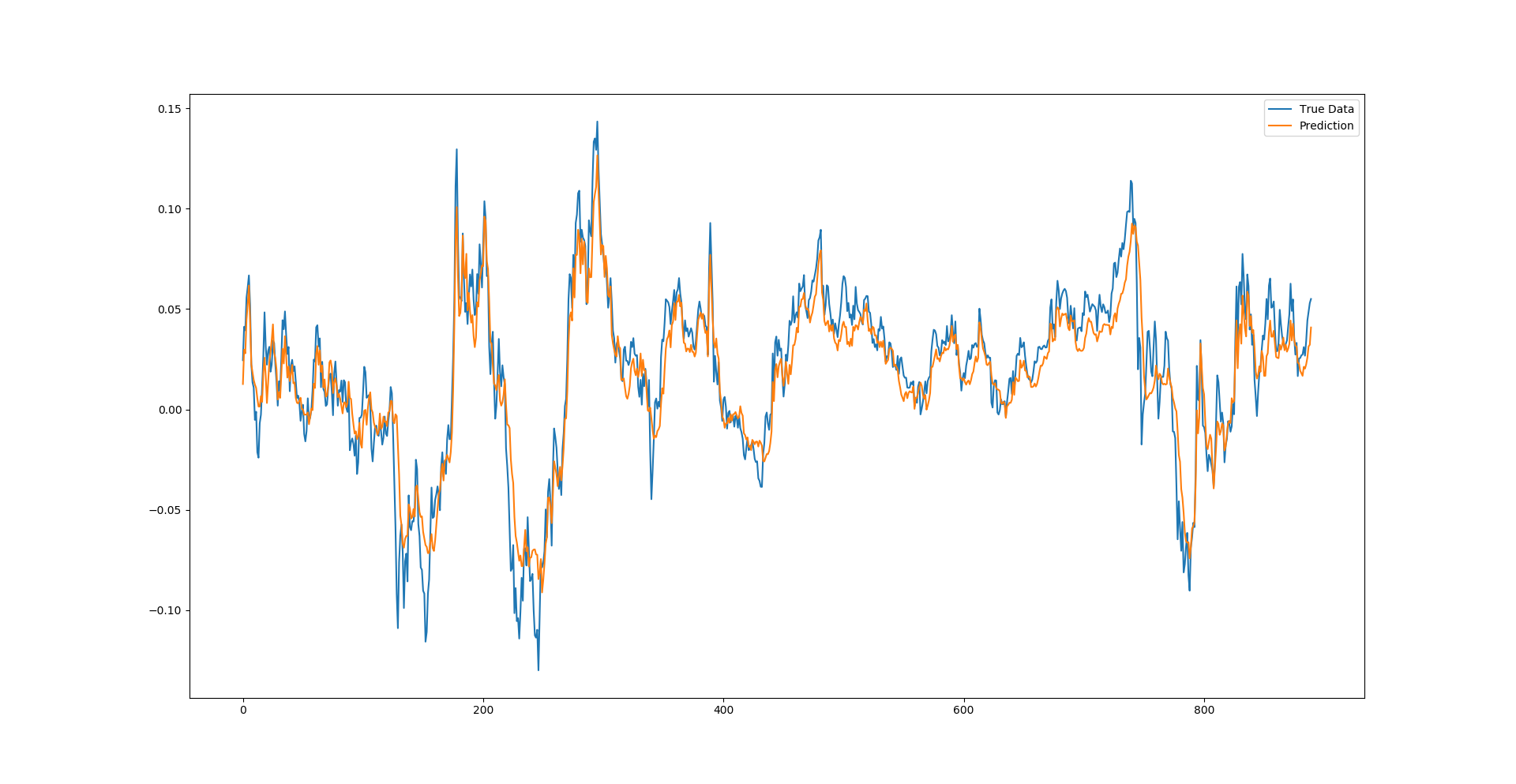S&P500 point-by-point prediction

Moving on to the full sequence prediction it seems like this proves to be the least useful prediction for this type of time series (at least trained on this model with these hyperparameters). We can see a slight bump on the start of the prediction where the model followed a momentum of some sorts, however very quickly we can see the model decided that the most optimal pattern was to converge onto some equilibrium of the time series. At this stage this might seem like it doesn't offer much value, however mean reversion traders might step in there to proclaim that the model is simply finding the mean that the price series will revert to when volatility is removed.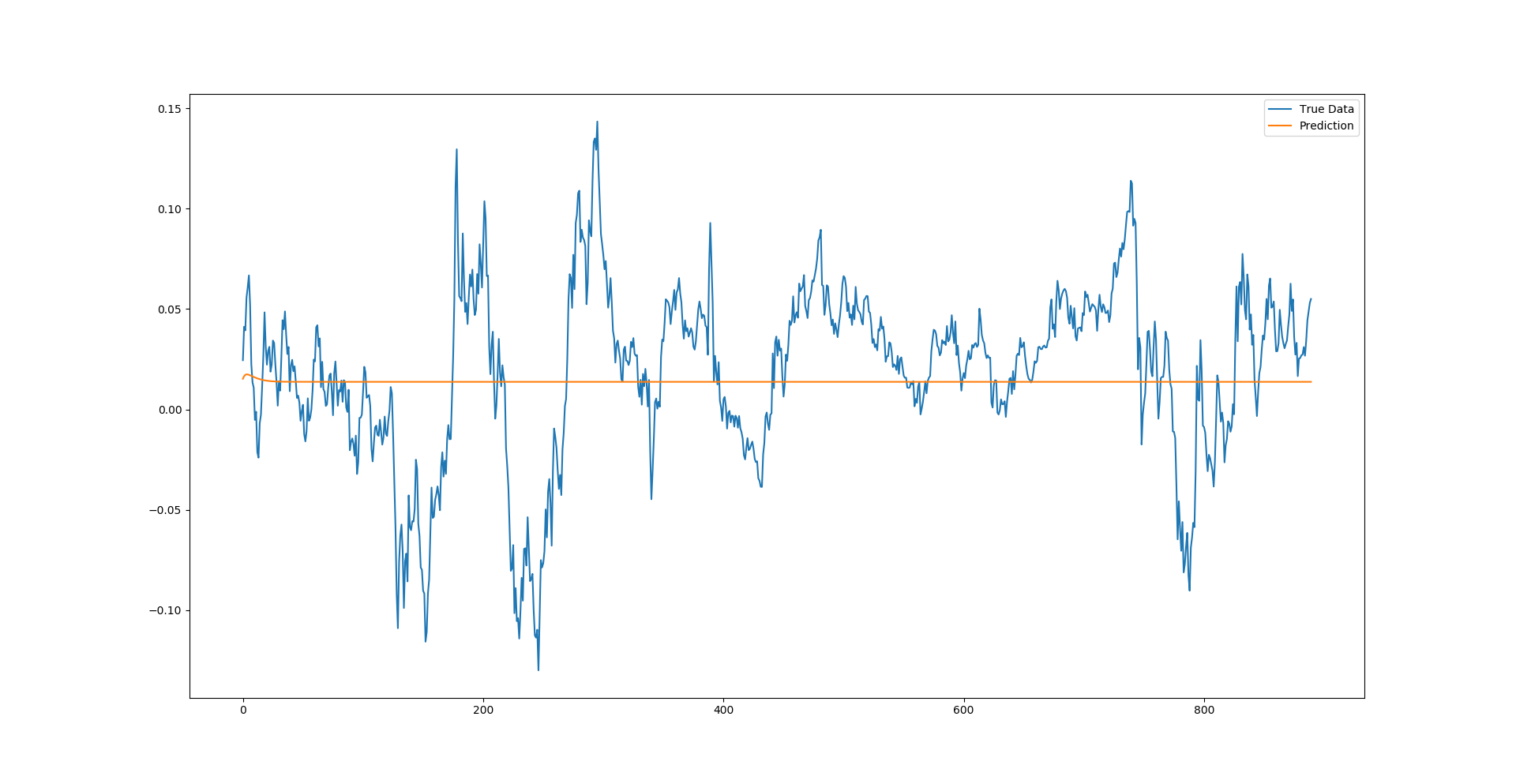S&P500 full sequence prediction

Lastly we have made a third type of prediction for this model, something I call a multi-sequence prediction. This is a blend of the full sequence prediction in the sense that it still initializes the testing window with test data, predicts the next point over that and makes a new window with the next point. However, once it reaches a point where the input window is made up fully of past predictions it stops, shifts forward one full window length, resets the window with the true test data, and starts the process again. In essence this gives multiple trend-line like predictions over the test data to be able to analyze how well the model can pick up future momentum trends.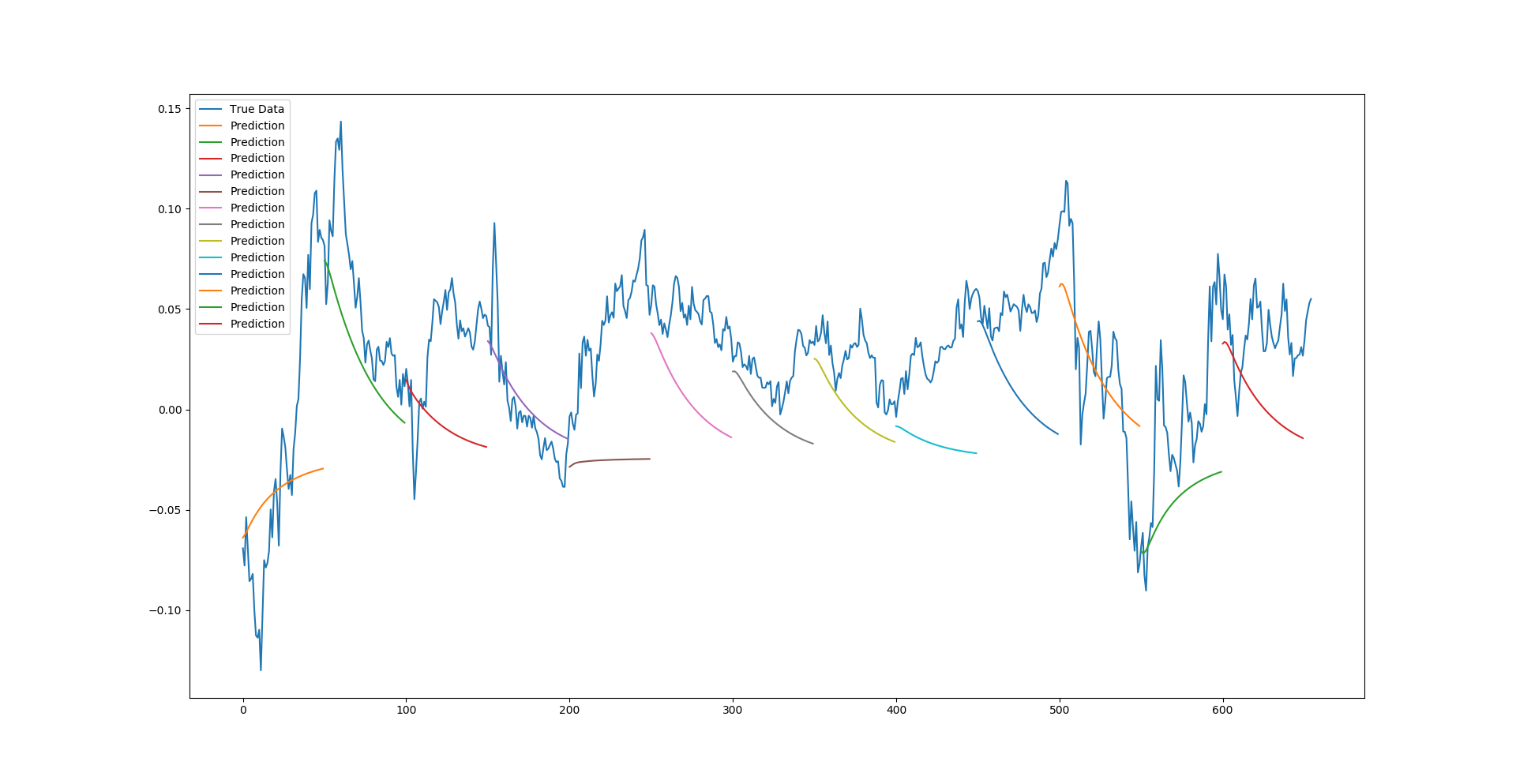S&P500 multi-sequence prediction

We can see from the multi-sequence predictions that the network does appear to be correctly predicting the trends (and amplitude of trends) for a good majority of the time series. Whilst not perfect, it does give an indication of the usefulness of LSTM deep neural networks in sequential and time series problems. Greater accuracy could most certainly be achieved with careful hyperparameter tuning.

## Multidimensional LSTM Prediction

So far our model has only taken in single dimensional inputs (the "Close" price in the case of our S&P500 dataset). But with more complex datasets there naturally exists many different dimensions for sequences which can be used to enhance the dataset and hence enhance the accuracy of our model.

In the case of our S&P500 dataset we can see we have Open, High, Low, Close and Volume that make up five possible dimensions. The framework we have developed allows for multi-dimensional input datasets to be used, so all we need to do to utilise this is to edit the columns and lstm first layer input_dim values appropriately to run our model. In this case I will run the model using two dimensions; "Close" and "Volume".

{
"data": {
"filename": "sp500.csv",
"columns": [
"Close",
"Volume"
],
"sequence_length": 50,
"train_test_split": 0.85,
"normalise": true
},
"training": {
"epochs": 1,
"batch_size": 32
},
"model": {
"loss": "mse",
"layers": [
{
"type": "lstm",
"neurons": 100,
"input_timesteps": 49,
"input_dim": 2,
"return_seq": true
},
{
"type": "dropout",
"rate": 0.2
},
{
"type": "lstm",
"neurons": 100,
"return_seq": true
},
{
"type": "lstm",
"neurons": 100,
"return_seq": false
},
{
"type": "dropout",
"rate": 0.2
},
{
"type": "dense",
"neurons": 1,
"activation": "linear"
}
]
}
}S&P500 multi-dimensional multi-sequence prediction using "Close" & "Volume"

We can see with the second "Volume" dimension added alongside the "Close" that the output prediction gets more granular. The predictor trend lines seem to have more accuracy in them to predict slight upcoming dips, not only the prevailing trend from the start and the accuracy of the trend lines also seems to improve in this particular case.

## Conclusion

Whilst this article aims to give a working example of LSTM deep neural networks in practice, it has only scratched the surface of their potential and application in sequential and temporal problems.

As of writing, LSTMs have been successfully used in a multitude of real-world problems from classical time series issues as described here, to text auto-correct, anomaly detection and fraud detection, to having a core in self-driving car technologies being developed.

There are currently some limitations with using the vanilla LSTMs described above, specifically in the use of a financial time series, the series itself has non-stationary properties which is very hard to model (although advancements have been made in using Bayesian Deep Neural Network methods for tackling non-stationarity of time series). Also for some applications it has also been found that newer advancements in attention based mechanisms for neural networks have out-performed LSTMs (and LSTMs coupled with these attention based mechanisms have outperformed either on their own).

As of now however, LSTMs provide significant advancements on more classical statistical time series approaches in being able to model the relationships non-linearly and being able to process data with multiple dimensions in a non-linear fashion.

The full source code of the framework we have developed can be found under an GNU General Public License (GPLv3) on the following GitHub page (we ask that credit is clearly attributed as "Jakob Aungiers, Altum Intelligence ltd" wherever this code is re-used): https://github.com/jaungiers/LSTM-Neural-Network-for-Time-Series-Prediction